Rotational Motion and Astrophysics Flashcards Preview

Linlithgow Academy Advanced Higher Physics > Rotational Motion and Astrophysics > Flashcards

Flashcards in Rotational Motion and Astrophysics Deck (57):
1

2

How would you find the instantaneous velocity of an object from a displacement-time graph such as this?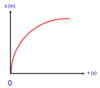3

How would you find the instantaneous acceleration of an object from a velocity-time graph such as this?4

How would you find the displacement of an object from a velocity-time graph such as this?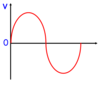Calculate the area underneath the graph- remember to include negative values.If the graph is a curve, the equation of the graph can be integrated to get an expression for displacement.5

6

What relationships would you use to convert between: Linear displacement and angular dispalcement Linear velocity and angular velocity Linear acceleration and angular acceleration

For any of these relationships, you would multiply the angular quantity by the radius of circular motion to get the linear quantity:7

How is angular velocity defined in terms of angular dispalcement?

Angular velocity is defined as the rate of change of angular displacement:8

How is angular acceleration defined in terms of angular velocity?

Angular acceleration is defined as the rate of change of angular velocity.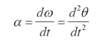9

10

11

12

13

14

15

16

17

18

19

20

21

22

The world-lines on the following space time diagram represent the motion of different objects, A, B and C.Describe the motion of objects A, B and C.23

24

25

26

27

28

29

When stars fuse hydrogen into helium via the proton-proton chain releasing energy (gamma rays) in the process, which two other particles are produced?

positrons and neutrinos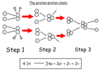30

In this simplified Hertzsprung-Russel diagram, which types of stars can be found in areas A, B, C and D?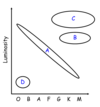31

32

33

34

35

36

A ball of weight W attached to a string is 'whirling' in a horizontal circle as shown below.What is the centripetal force acting on the ball?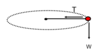37

A ball of weight W attached to a string under tension T is 'whirling' in a vertical circle as shown below.Determine a relationship for the centripetal force acting on the ball: at the bottom. at the sides. at the top.38

A 'conical pedulum' is shown below.In terms of the components of tension in the string determine expressions for the: Centripetal force Weight of the 'bob'.Centripetal force = T sinθ Weight = T cosθ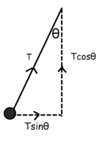39

40

41

42

43

44

45

46

47

48

49

Shown is the relationship used to find the gravitational potential at a point in space.How could you find the gravitational potential energy of a mass, m, at that point?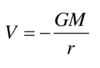Multiply the gravitational potential by the mass:Ep = Vm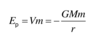50

Derive the expression shown below for escape velocity.51

52

53

54

The following experimental setup can be used to determine the moment of inertia of a disc which is allowed to rotate on a frictionless air bearing turntable.Explain the measurments which will need to be taken, and how to use these to determine the moment of inertia of the disc.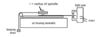Find the torque applied to the disc by using the relationship T = Fr.Use the light gate to measure the angular acceleration of the disc.Vary the hanging mass to vary the torque, and repeat a few times.Plot T against α, and take the gradient of the straight line which will be equal to the monemt of inertia of the disc.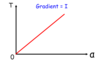55

An ice skater is spinning with his arms outward as shown.He then pulls his arms inward.Explain what happens to his angular momentum and rotational kinetic energy.56

57